### Home > INT2 > Chapter 12 > Lesson 12.1.2 > Problem12-26

12-26.

If f(x) = x2, write an equation for each of the following transformations, then sketch a graph and write a description of the transformation. Homework Help ✎

 a. y = –f(x) b. y = f(x – 3) c. y = f(x) – 3 d. y = f(2x)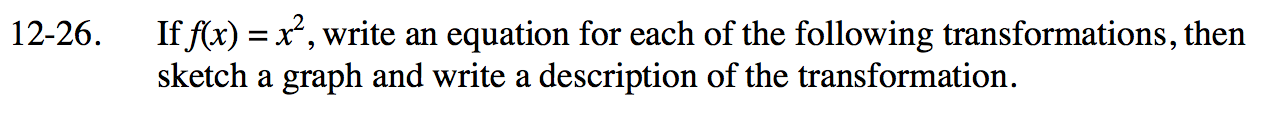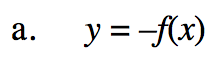y = −f(x) = −x2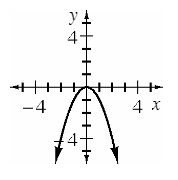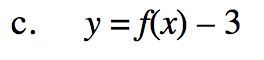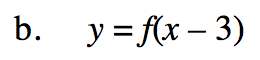y = f(x − 3) = (x − 3)2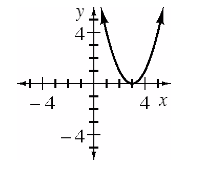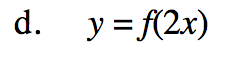Use the same method as (a-b).